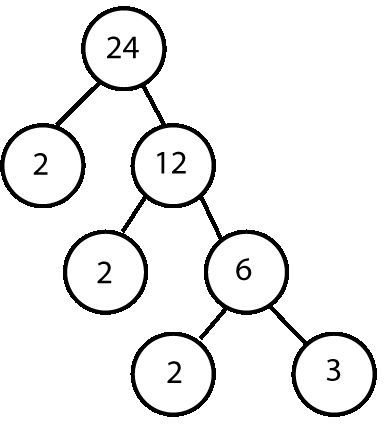# Factor Trees in Math: Making Factoring Easy

There are many times when we need to know the factors of a number. For example, when adding, subtracting, or comparing fractions, you first need to find a common denominator. One way you can do this is to check every number up to half the number you’re factoring and see if it divides into your original number. While this works, it will obviously take a long time for large numbers! Fortunately, there is a better way. Let’s learn about using factor trees in math.

A factor tree lets you find all of the prime factors of your number, where a prime factor is a prime number which evenly divides into your original number. It also tells you the number of times that each prime factor appears. For example, suppose you want to factor the number 24. Rather than getting the factors 1, 2, 3, 4, 6, 8, 12, and 24, you’ll just get 2 x 2 x 2 x 3 = 24, or 23 x 3 = 24, Notice that every composite factor is the product of two or more prime factors.

## Building the Factor TreeSo let’s go through the process of creating the factor tree for the number 24. To start, write down 24 on a piece of paper (leave plenty of room on either side) and circle it. The circle shows that this is actually the node of a tree; lines between nodes will are called edges of the tree.

The first prime number is two. Two divides evenly into 24 (that is, 24 divided by 2 has no remainder), so write two underneath 24 and to the left. 24 divided by 2 is 12, so write 12 to the right of the two. Circle both numbers and draw an edge from each new circle up to the circle containing the 24. Your drawing should look like the top section of the picture at right.

Now, we go down to the 12. Twelve is also divisible by two, so we put another two underneath it, along with a six, which is the quotient of twelve and two. Six is also divisible by two, so underneath the six we put a two and a three.

Three is not divisible by two, which means we need to try the next prime number. The smallest prime number after two is three; in other words, the current value is already prime, so we’re done. Since the current node (each circled number is called a node of the tree) is prime, every leaf (a node with no children, that is, no nodes hanging from it) of the tree is prime, and we are done. By reading off all the leaves, we can see that 24 = 2 x 2 x 2 x 3.

## Why Use This Methodology?

As you can see, building factor trees in math is pretty easy. All you have to do is look at a series of increasingly smaller numbers and find the smallest prime (which is also the smallest integer) that number is divisible by.

When we finish, as long as we did all of the division problems correctly, we know that we have all of the prime factors. If for some reason we need all of the factors, we simply find all the ways to multiply the prime factors together. Thus, factor trees make math problems that require factoring very easy!